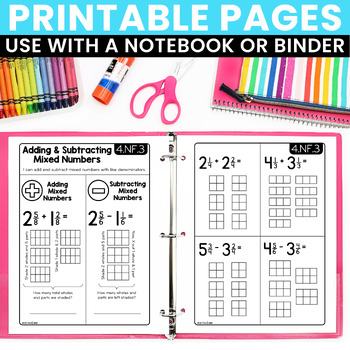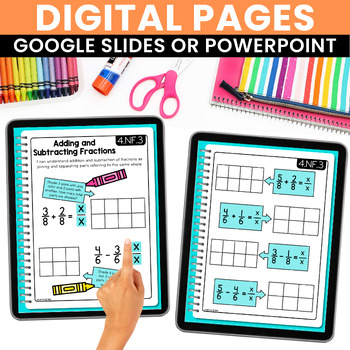# Math Interactive Notebook 4th Grade Fractions

Rated 4.87 out of 5, based on 3288 reviews
3.3k Ratings;
3rd - 5th, Homeschool
Subjects
Resource Type
Standards
Formats Included
• PDF
Pages
38 pages
Report this resource to TPT

#### What educators are saying

I taught math for the first time last year and this was a life-saver! Such a great resource for students!
I used this unit for differentiated instruction in my resource classroom. It is amazing! I appreciated the visuals and how user friendly these notebooks are!
##### Also included in
1. What are Interactive Math Notebooks?Interactive math notebooks are teaching pages used to introduce and teach math concepts and skills. Each set of notebook pages includes definitions, explanations, rules, and algorithms that may be needed to address each standard. Practice problems are included to
Price \$26.50Original Price \$33.00Save \$6.50
2. This BUNDLE includes everything you need for 4th grade math!!★ Interactive Notebook Pages★ Math Quick Checks ★ Math Menus (Perfect for homework or early finishers!!)★ Open-Ended Math Pages★ Math Mastery Task Cards★ Units for Fractions, Decimals, Measurement &amp; GeometryFeel free to email with any
Price \$82.50Original Price \$103.70Save \$21.20
3. This BUNDLE includes everything you need for 4th &amp; 5th grade math!!★ Interactive Notebook Pages★ Math Quick Checks★ Math Menus (Perfect for homework or early finishers!!)★ Open-Ended Math Pages★ Math Exit Slips★ Math Mastery Task Cards★ Printables for Fractions &amp; Decimals★ Plus, MORE!!Feel f
Price \$113.50Original Price \$162.15Save \$48.65
4. This custom bundle includes math notebooks for grades 2nd to 5th.If you're interested in your own custom bundle, feel free to email me at rebecca@createteachshare.com.
Price \$56.25Original Price \$94.00Save \$37.75
5. This BUNDLE includes everything you need for 3rd to 5th grade math!!★ Interactive Notebook Pages★ Math Quick Checks★ Math Menus (Perfect for homework or early finishers!!)★ Open-Ended Math Pages★ Math Exit Slips★ Math Mastery Task Cards★ Printables for Fractions &amp; Decimals★ Plus, MORE!!Feel free
Price \$139.50Original Price \$223.10Save \$83.60
6. This BUNDLE includes everything you need for 3rd to 4th grade math!!★ Interactive Notebook Pages★ Math Quick Checks★ Math Menus (Perfect for homework or early finishers!!)★ Open-Ended Math Pages★ Math Exit Slips★ Math Mastery Task Cards★ Printables for Fractions, Decimals, Measurement, and Geometry★
Price \$115.25Original Price \$164.65Save \$49.40
7. This BUNDLE includes everything you need for 2nd to 5th grade math!!★ Interactive Notebook Pages★ Math Quick Checks★ Math Menus (Perfect for homework or early finishers!!)★ Open-Ended Math Pages★ Math Exit Slips★ Math Mastery Task Cards★ Printables for Fractions, Decimals, Measurement, and Geometry★
Price \$152.95Original Price \$277.80Save \$124.85

### Description

What are Interactive Math Notebooks?

Interactive math notebooks are teaching pages used to introduce and teach math concepts and skills. Each set of notebook pages includes definitions, explanations, rules, and algorithms that may be needed to address each standard. Practice problems are included to be used for guided practice. Once completed, these pages serve as a great reference tool for students to refer back throughout the year.

How do I use them with my students?

Notebook pages can be used to introduce, reinforce, or review math standards. They can be used for direct instruction for a whole class or in small groups. For the printable version, students first cut out the pages and glue them into a spiral notebook. With teacher guidance, students take notes and work out practice problems. Students can then highlight or add color to their notebook pages. The digital pages include the same content, but require no cutting or pasting!

What materials are needed for printable notebook pages?

A spiral notebook for each student, scissors, glue sticks, pencils, and colored pencils, crayons, markers (optional)

What is included in this resource?

• Notebook pages for each standard. Each concept or skill includes a double-page spread. (Printable & Digital)
• Answer Key (Available with printable pages)

What standards are covered?

• Equivalent Fractions (4.NF.1)
• Comparing Fractions (4.NF.2)
• Decomposing Fractions (4.NF.3)
• Adding & Subtracting Fractions (4.NF.3)
• Adding & Subtracting Mixed Numbers (4.NF.3)
• Word Problems: Adding & Subtracting Fractions (4.NF.3)
• Multiplying Fractions by Whole Numbers (4.NF.4)
• Word Problems: Multiplying Fractions by Whole Numbers (4.NF.4)
• Fractions: Denominators of 10 and 100 (4.NF.5)
• Relating Fractions and Decimals (4.NF.6)
• Comparing Decimals (4.NF.7)

★★This resource is included in myULTIMATE 4th Grade Math Bundle!!★★

Check out the rest of my Math Activities for 4th Grade:

4th Grade Interactive Math Notebook: OA

4th Grade Interactive Math Notebook: NBT

4th Grade Interactive Math Notebook: NF

4th Grade Interactive Math Notebook: MD

4th Grade Interactive Math Notebook: G

Common Core Math Quick Checks

Math Exit Slips

Solve & Sort Math Centers

Total Pages
38 pages
Included
Teaching Duration
Other
Report this resource to TPT
Reported resources will be reviewed by our team. Report this resource to let us know if this resource violates TPT’s content guidelines.

### Standards

to see state-specific standards (only available in the US).
Explain why a fraction 𝘢/𝘣 is equivalent to a fraction (𝘯 × 𝘢)/(𝘯 × 𝘣) by using visual fraction models, with attention to how the number and size of the parts differ even though the two fractions themselves are the same size. Use this principle to recognize and generate equivalent fractions.
Compare two fractions with different numerators and different denominators, e.g., by creating common denominators or numerators, or by comparing to a benchmark fraction such as 1/2. Recognize that comparisons are valid only when the two fractions refer to the same whole. Record the results of comparisons with symbols >, =, or <, and justify the conclusions, e.g., by using a visual fraction model.
Understand a fraction 𝘢/𝘣 with 𝘢 > 1 as a sum of fractions 1/𝘣.
Understand addition and subtraction of fractions as joining and separating parts referring to the same whole.
Decompose a fraction into a sum of fractions with the same denominator in more than one way, recording each decomposition by an equation. Justify decompositions, e.g., by using a visual fraction model. Examples: 3/8 = 1/8 + 1/8 + 1/8; 3/8 = 1/8 + 2/8; 2 1/8 = 1 + 1 + 1/8 = 8/8 + 8/8 + 1/8.